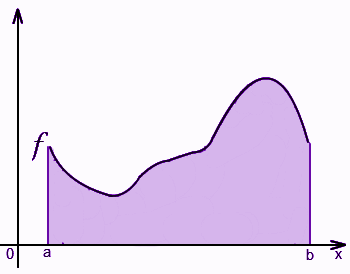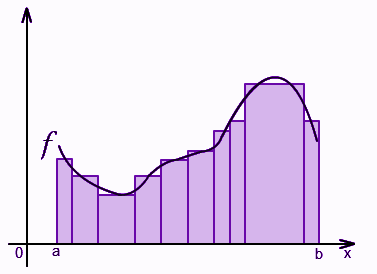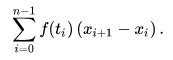Checkout JEE MAINS 2022 Question Paper Analysis : Checkout JEE MAINS 2022 Question Paper Analysis :

# Riemann Integral

In real analysis, Riemann Integral, developed by the mathematician Bernhard Riemann, was the first accurate definition of the integral of a function on an interval. The real analysis is a very important and a vast branch of Mathematics, applied in higher studies. It was introduced for the study of the theory of functions for real variables. It deals with real variables, real numbers and real-valued functions. We can also say that it studies analytic properties of sequences and real functions. It includes the study of limits and convergence of real-number sequences. This analysis deals with continuity and other properties of real-valued functions. It is also concerned with the calculus over real numbers.

Riemann integral is applied to many practical applications and functions. It can be measured and approximated by the numerical integration and by the fundamental theorem of calculus. It is defined as a definite integral in calculus, used by engineers and physicists. Let us learn more here.

## Riemann Integral Definition

Let us suppose that f is a function, defined over a closed interval represented by [a, b]. Suppose f is a non-negative and continuous function. The integral of f with respect to x signifies the area between the graph of f and X-axis. This area would be called definite integral of a function f from a to b.The Riemannian method of determining this area (or definite integral) is: “if the area is divided into rectangles of very small breadth, then it would be simpler to calculate the area of such rectangles and then we can add them up”. See the following diagram;It is quite apparent that if these rectangles are very narrow, then the approximation error would be negligibly small. For the narrower rectangles, the measured approximated area may converge to a number which is called the area under the curve of the function f. If we do not draw tiny rectangles, then this method tends to break down. To make Riemann procedure accurate, we may determine the widths of rectangles by splitting the given interval [a, b] into smaller parts.

## Riemann Sums

Let us understand a few other concepts before learning about the Riemann sum.

Partition:

Suppose a closed interval [a, b] ∈ R.

The partition of this interval is said to be a sequence of the following form:

a = x0 < x1 < x2 < … < xn

where, every [xi,xi+1 ] is known as a sub-interval.

Norm:

The length of the biggest sub-interval is called a norm or a mesh, i.e. norm = max

Tagged Partition:

For interval [a, b], a partition P(x, t) along with a sequence of finite numbers t0,t1,…,tn-1 is known as tagged partition if it satisfies the condition that, ti ∈ [xi, xi+1] for every i.

Riemann Sum:

The Riemann sum of a real-valued function f on the interval [a, b] is defined as the sum of f with respect to the tagged partition of [a, b]. I.e.Every term in Riemann sum denotes the area of a rectangle having length or height f(ti) and breadth xi+1−xi. Hence, the Riemann sum gives the area of all the rectangles and thus the area under the curve within the interval [a, b] or definite integral.

## Riemann Integral Formula

Let f be a real-valued function over the interval [a, b] and let L be a real number. Then, f is called integrable within [a, b] if and only if there is a δ > 0 for each ϵ > 0 such that for each partition having a property that ||P|| < δ, we can have;

 |S(f,P)−L| < ϵ

Where, L is known as the integral of f over the interval [a, b], thus we write it as below :

L = ∫ab f(x) dx

## Properties of Riemann Integral

There are basically three major properties:

1. Linearity
2. Monotonicity

### Linearity

If f : [a, b] → R is integrable and c ∈ R, then cf is integrable and

ab cf = c ∫ab f

And also,

ab (f + g) = ∫ab f + ∫ab g

### Monotonicity

As per this property, if f ≤ g, then;

ab f ≤ ∫ab g

If a < c < b, then;

ac f + ∫cb f = ∫ab f

## Applications

The Riemann integral is used in many fields, such as;

• In integration as well as differential calculus
• They are applied from calculus to physics problems
• Used in partial differential equations and representation of functions by trigonometric series
• It is also used for the measurement of distance travelled by some object, because we can easily retrieve the average velocity of the journey and total time by the Velocity versus Time graph. The distance travelled is represented by the area under the given curve

Although having such huge applications, the Riemann integral is quite challenging to handle as we can see that its definition is little sophisticated. So, it is a bit inconvenient to use Riemann integral in practical life.